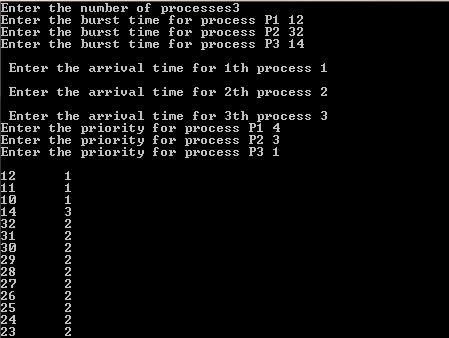# C / C++ Code / Program For Priority Scheduling, And Calculating The Average Waiting Time Of Each Process

/*The Following C / C++ Code / Program For Priority Scheduling, And Calculating The Average Waiting Time Of Each Process Ask the user about the number of processes & then the burst time of each process individually. Then after it the arrival time for each process & at last the priority value of each process. Lower the priority number higher is the priority. The output is the average wait time of each process  */

/* Below is the code for Priority Scheduling */

#include<iostream>
#include<conio.h>
using namespace std;
void main()
{
int i,j,Wt,B,A,Tt=0,temp,n,Bu,Pr;
char S;
float Twt=0.0,Awt;
cout<<"Enter the number of processes";
cin>>n;
for(i=1;i<=n;i++)
{
cout<<"Enter the burst time for process P"<<i<<" ";
cin>>Bu[i];
}
for(i=1;i<=n;i++)
{
B[i]=Bu[i];
Wt[i]=0;
S[i]='T';
Tt=Tt+B[i];
cout<<"\n Enter the arrival time for "<<i<<"th process ";
cin>>A[i];
}
for(i=1;i<=n;i++)
{
cout<<"Enter the priority for process P"<<i<<" ";
cin>>Pr[i];
}
int flag=0,t=0;
temp=i=1;
while(t<Tt)
{
cout<<"\n"<<B[i]<<"\t"<<i;
if(temp!=i)
{
Wt[i]=t-(Bu[i]-B[i]);
temp=i;
}
B[i]=B[i]-1;
if(B[i]==0)
S[i]='F';
t=t+1;
if(S[i]!='F')
{
j=1;
while(j<=n)
{
if(S[j]!='F' && A[j]<=t && i!=j &&Pr[j]==i)
{
i=j;
}
else
j++;
}
}
else
{
i++;
if(i>n)
i=1;
}
}
for(i=1;i<=n;i++)
{
cout<<"\nWaiting time for process P "<<i<<" ="<<(Wt[i]-A[i]);
Twt+=(Wt[i]-A[i]);
}
Awt=Twt/n;
cout<<"\n The average waiting time is "<<Awt;
getche();
}

Screenshot:Priority Scheduling & wait time calculation Through C++ CodePriority Scheduling & wait time calculation Through C++ Code